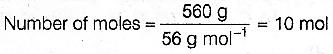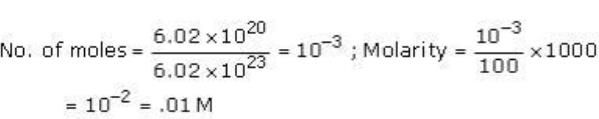Courses

# Test: Previous Year Questions: Stoichiometry

## 6 Questions MCQ Test Chemistry Class 11 | Test: Previous Year Questions: Stoichiometry

Description
This mock test of Test: Previous Year Questions: Stoichiometry for JEE helps you for every JEE entrance exam. This contains 6 Multiple Choice Questions for JEE Test: Previous Year Questions: Stoichiometry (mcq) to study with solutions a complete question bank. The solved questions answers in this Test: Previous Year Questions: Stoichiometry quiz give you a good mix of easy questions and tough questions. JEE students definitely take this Test: Previous Year Questions: Stoichiometry exercise for a better result in the exam. You can find other Test: Previous Year Questions: Stoichiometry extra questions, long questions & short questions for JEE on EduRev as well by searching above.
QUESTION: 1

### The weight of 2.01 × 1023 molecules of CO is-   [AIEEE-2002]

Solution:

No. of molecules = mass / molecular mass * Na . so according to the formula 2.01*10^23=mass /28 * 6.022*10^23 = mass =2.01*10^23 *28 /6.022 *10^23 = 9.3gm

QUESTION: 2

### In an organic compound of molar mass 108 gm mol-1 C, H and N atoms are present in 9 : 1 : 3.5 by weight. Molecular formula can be  [AIEEE-2002]

Solution:

Mass of 1 mole of compound = 108g.

Let the mass of C in 1 mole of compound = 9x.

mass of H in 1 mole of compound = x

mass of N in 1 mole of compound = 3.5x

therefore total mass = 9x+x+3.5x = 13.5x.

According to the question

13.5x=108

=> x=108/13.5 = 8.

mass of C=9x=72g = 12(6)

mass of H= x= 8g = 1(8)

mass of N=3.5x=28g = 14(2)

molar mass of C,H,N = 12, 1, 14.

therefore there are 6, 8, 2 molecules of C,H,N

MOLECULAR FORMULA = C6H8N2.

QUESTION: 3

### Number of atoms in 560 gm of Fe (atomic mass 56 g mol-1) is -   [AIEEE-2003]

Solution:

560 g of FeFor 70 g of N
14 g N = 1 mol of N -atom
70 g N = 5 mol of N -atom

For 20 g of H
1 g H = 1 mol of H.-atom
20 g H = 20 mol of H-atom

QUESTION: 4

6.02 ×1020 molecules of urea are present in 100 ml of its solution. The concentration of urea solution is -

(Avogadro constant, NA = 6.02 ×1023 mol-1)

[AIEEE-2004]

Solution:QUESTION: 5

How many moles of magnesium phosphate, Mg3(PO4)2 will contain 0.25 mole of oxygen atoms ?

[AIEEE 2006]

Solution:

1mole of Mg3 (PO4 )2
=> 3moles of Mg atom + 2 moles of P atom + 8 moles of O atom
8 moles of oxygen atoms are present in = 1mole of Mg3 (PO4)2
0.25mole of oxygen atoms are present in = (1 x 0.25)/8
= 3.125 × 10–2moles of Mg3 (PO4 ) 2.

QUESTION: 6

In the reaction,

2Al(s)+6HCl(aq) → 2Al 3+(aq)+6Cl-(aq)+3H2 (g),

[AIEEE 2007]

Solution:

Explanation : 6 moles of HCl reacts with 3 moles of hydrogen.

Hence, 1 mole of HCl will react with 3/6

= 0.5 moles of hydrogen.

At STP, 1 mole of hydrogen corresponds to 22.4 L.

Hence, 0.5 moles of hydrogen will correspond to 22.4/2 = 11.2L.

Thus, 11.2 L of hydrogen gas at STP is produced for every mole of HCl consumed.